### Practice Set 3.1 Circle Class 10th Mathematics Part 2 MHB Solution

Practice Set 3.1

1. In the adjoining figure the radius of a circle with centre C is 6 cm, line AB is a…
2. In the adjoining figure, O is the centre of the circle. From point R, seg RM and seg RN…
3. Seg RM and seg RN are tangent segments of a circle with centre O. Prove that seg OR…
4. What is the distance between two parallel tangents of a circle having radius4.5 cm ?…

###### Practice Set 3.1
Question 1.

In the adjoining figure the radius of a circle with centre C is 6 cm, line AB is a tangent at A. Answer the following questions.

(1) What is the measure of ∠CAB ? Why?

(2) What is the distance of point C from line AB? Why?

(3) d(A,B) = 6 cm, find d(B,C).

(4) What is the measure of ∠ABC ? Why?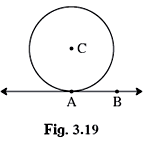(1) ere CA is the radius of the circle and A is the point of contact of the tangent AB.

⇒ ∠CAB = 90° Using tangent-radius theorem which states that a tangent at any point of a circle is perpendicular to the radius at the point of contact.

(2) CA is the radius of the circle which is perpendicular to the tangent AB.

So, the perpendicular distance of line AB from C = CA = 6 cm

(3) In triangle ABC right-angled at A,

Given AB = 6 cm and CA = 6 cm

BC2 = AB2 + CA2 {Using Pythagoras theorem}

⇒ BC2 = 62 + 62

⇒ BC2 = 36 + 36

⇒ BC = √72

⇒ BC = 6√2 cm

(4) In triangle ABC right-angled at A,

AB = CA = 6 cm

⇒∠ABC = ∠ACB {Angles opposite to equal sides are equal}

⇒∠ABC + ∠ACB + ∠ BAC = 180° {Angle sum property of the triangle}

⇒ 2∠ABC = 90° {∵ ∠ BAC = 90°}

⇒ ∠ABC = 45°

Question 2.

In the adjoining figure, O is the centre of the circle. From point R, seg RM and seg RN are tangent segments touching the circle at M and N. If (OR) = 10 cm and radius of the circle = 5 cm, then

(1) What is the length of each tangent segment?

(2) What is the measure of ∠MRO?

(3) What is the measure of ∠MRN?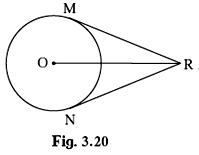(1) Here OM is the radius of the circle and M and N are the points of contact of MR and NR respectively.

⇒ ∠RMO = 90° Using tangent-radius theorem which states that a tangent at any point of a circle is perpendicular to the radius at the point of contact.

In triangle ORM right-angled at M,

Given that OR = 10 cm and OM = 5 cm {Radius of the circle}

OR2 = OM2 + RM2 {Using Pythagoras theorem}

⇒MR2 = 102 -52

⇒MR2 = 100 - 25

⇒ MR = √75

⇒ MR = 5√3 cm

Also, RN = 5√3 cm {∵ Tangents from the same external point are congruent to each other.}

(2)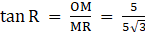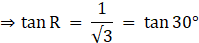⇒∠MRO = 30°

(3) Similarly, ∠NRO = 30°

⇒∠MRN = ∠ MRO + ∠NRO = 30° + 30° = 60°

Question 3.

Seg RM and seg RN are tangent segments of a circle with centre O. Prove that seg OR bisects ∠MRN as well as ∠MON.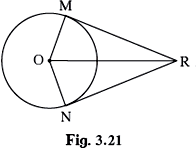In triangle MOR and triangle NOR,

MR = NR {∵Tangents from same external point are congruent to each other.}

OR = OR {Common}

OM = ON {Radius of the circle}

⇒ ΔMOR ≅ ΔNOR {By SSS}

⇒ ∠ROM = ∠RON

And ∠MRO = ∠NRO {C.P.C.T.}

Hence proved that seg OR bisects ∠MRNas well as ∠MON.

Question 4.

What is the distance between two parallel tangents of a circle having radius4.5 cm ? Justify your answer.

Let BC and DE be the parallel tangents to a circle centered at A with point of contact O and H respectively. On joining OH, we find OH is the diameter of the circle.∠ BOA = 90° = ∠ DHA {Using tangent-radius theorem which states that a tangent at any point of a circle is perpendicular to the radius at the point of contact.}

Distance between BC and DE = OH

∵ OH is perpendicular to BC and DE.

OH = 2 × 4.5 cm = 9 cm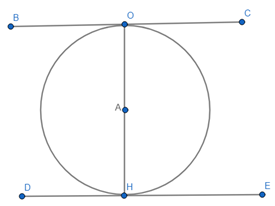## PDF FILE TO YOUR EMAIL IMMEDIATELY PURCHASE NOTES & PAPER SOLUTION. @ Rs. 50/- each (GST extra)

SUBJECTS

HINDI ENTIRE PAPER SOLUTION

MARATHI PAPER SOLUTION
SSC MATHS I PAPER SOLUTION
SSC MATHS II PAPER SOLUTION
SSC SCIENCE I PAPER SOLUTION
SSC SCIENCE II PAPER SOLUTION
SSC ENGLISH PAPER SOLUTION
SSC & HSC ENGLISH WRITING SKILL
HSC ACCOUNTS NOTES
HSC OCM NOTES
HSC ECONOMICS NOTES
HSC SECRETARIAL PRACTICE NOTES

2019 Board Paper Solution

HSC ENGLISH SET A 2019 21st February, 2019

HSC ENGLISH SET B 2019 21st February, 2019

HSC ENGLISH SET C 2019 21st February, 2019

HSC ENGLISH SET D 2019 21st February, 2019

SECRETARIAL PRACTICE (S.P) 2019 25th February, 2019

HSC XII PHYSICS 2019 25th February, 2019

CHEMISTRY XII HSC SOLUTION 27th, February, 2019

OCM PAPER SOLUTION 2019 27th, February, 2019

HSC MATHS PAPER SOLUTION COMMERCE, 2nd March, 2019

HSC MATHS PAPER SOLUTION SCIENCE 2nd, March, 2019

SSC ENGLISH STD 10 5TH MARCH, 2019.

HSC XII ACCOUNTS 2019 6th March, 2019

HSC XII BIOLOGY 2019 6TH March, 2019

HSC XII ECONOMICS 9Th March 2019

SSC Maths I March 2019 Solution 10th Standard11th, March, 2019

SSC MATHS II MARCH 2019 SOLUTION 10TH STD.13th March, 2019

SSC SCIENCE I MARCH 2019 SOLUTION 10TH STD. 15th March, 2019.

SSC SCIENCE II MARCH 2019 SOLUTION 10TH STD. 18th March, 2019.

SSC SOCIAL SCIENCE I MARCH 2019 SOLUTION20th March, 2019

SSC SOCIAL SCIENCE II MARCH 2019 SOLUTION, 22nd March, 2019

XII CBSE - BOARD - MARCH - 2019 ENGLISH - QP + SOLUTIONS, 2nd March, 2019

HSC Maharashtra Board Papers 2020

(Std 12th English Medium)

HSC ECONOMICS MARCH 2020

HSC OCM MARCH 2020

HSC ACCOUNTS MARCH 2020

HSC S.P. MARCH 2020

HSC ENGLISH MARCH 2020

HSC HINDI MARCH 2020

HSC MARATHI MARCH 2020

HSC MATHS MARCH 2020

SSC Maharashtra Board Papers 2020

(Std 10th English Medium)

English MARCH 2020

HindI MARCH 2020

Hindi (Composite) MARCH 2020

Marathi MARCH 2020

Mathematics (Paper 1) MARCH 2020

Mathematics (Paper 2) MARCH 2020

Sanskrit MARCH 2020

Important-formula

THANKS